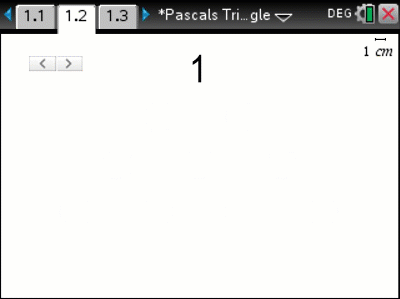# Activities

•• ##### AuthorAust Senior

50 Minutes

• ##### Device
•TI-Nspire™ CX CAS
• ##### Software

TI-Nspire™ CAS

4.5

## Pascals Triangle Hidden Gems#### Activity Overview

Pascal’s Triangle goes way beyond coefficients of binomial expansions and combinatorics. In this activity students are introduced to these basics but go on to explore other relationships and patterns such as the triangular and tetrahedral numbers, the Fibonacci sequence and even Euler’s number. There are so many hidden gems in this amazing triangle.

#### Objectives

By exploring Pascal’s triangle in greater depth, students are more likely to remember this amazing triangle. The numeric and algebraic applications will help improve students ‘by-hand’ algebraic skills beyond basic simplification.

#### Vocabulary

• Pascal
• Combinatorics
• Binomial Expansion
• Triangular Numbers
• Tetrahedral Numbers
• Fibonacci
• Euler
• Summation (Sigma Notation)
• Product (Pi Notation)